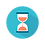From: Bayesian Models for Astrophysical Data, Cambridge Univ. Press

(c) 2017,  Joseph M. Hilbe, Rafael S. de Souza and Emille E. O. Ishida

you are kindly asked to include the complete citation if you used this material in a publication

Code 5.14 Beta model in Python using Stan

================================================
import numpy as np
import statsmodels.api as sm
import pystan

from scipy.stats import uniform
from scipy.stats import beta as beta_dist

# Data
np.random.seed(1056)                                          # set seed to replicate example
nobs= 2000                                                           # number of obs in model
x1 = uniform.rvs(size=nobs)                                # random uniform variable

beta0 = 0.3
beta1 = 1.5
theta = 15

xb = beta0 + beta1 * x1
exb = np.exp(-xb)
p = exb / (1 + exb)

y = beta_dist.rvs(theta * (1 - p), theta * p)           # create y as adjusted

# Fit
mydata = {}
mydata['N'] = nobs                                               # sample size
mydata['x1'] = x1                                                 # predictors
mydata['y'] = y                                                     # response variable

stan_code = """
data{
int<lower=0> N;
vector[N] x1;
vector<lower=0, upper=1>[N] y;
}
parameters{
real beta0;
real beta1;
real<lower=0> theta;
}
model{
vector[N] eta;
vector[N] p;
vector[N] shape1;
vector[N] shape2;

for (i in 1:N){
eta[i] = beta0 + beta1 * x1[i];
p[i] = inv_logit(eta[i]);
shape1[i] = theta * p[i];
shape2[i] = theta * (1 - p[i]);
}

y ~ beta(shape1, shape2);
}
"""

# Run mcmc
fit = pystan.stan(model_code=stan_code, data=mydata, iter=5000, chains=3,
warmup=2500, n_jobs=3)

# Output
print(fit)

================================================Output on screen:

Inference for Stan model: anon_model_955d748487258410d89e68cefe178077.
3 chains, each with iter=5000; warmup=2500; thin=1;
post-warmup draws per chain=2500, total post-warmup draws=7500.

mean    se_mean         sd        2.5%           25%          50%           75%        97.5%       n_eff     Rhat
beta0         0.34        4.2e-4      0.02         0.29           0.32           0.34           0.35           0.38     3132.0       1.0
beta1         1.43        7.8e-4      0.04         1.35           1.40           1.43           1.46           1.52     3160.0       1.0
theta        15.10        7.3e-3      0.45       14.20         14.80         15.10         15.40         16.00     3906.0       1.0
lp__     1709.40           0.02      1.16     1706.5     1708.80     1709.60     1710.20     1710.70     3033.0       1.0

Samples were drawn using NUTS at Thu Dec 22 17:35:40 2016.
For each parameter, n_eff is a crude measure of effective sample size,
and Rhat is the potential scale reduction factor on split chains (at
convergence, Rhat=1).